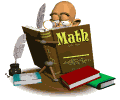• #Math always keeps us busy.  Many students are using basic skills such as; number recognition, counting, making sets, and writing numbers to use in higher level thinking problems.  We use lots of different ways to introduce and teach concepts.  We use manipulatives and we act out problems.  We also draw problems when we are adding and subtracting.

Key concepts that will be covered are Common Core Standards.  Sometimes you may see modifications to the lesson or other ways to help students solve the problem and understand the concept.  Some of the concepts and standards are:

Addition and Subtraction with known parts and unknown parts, Measurement, Double digit addition with and without regrouping, single digit addition and subtraction, number recognition and matching numbers to sets.

http://www.playkidsgames.com/games/mathfact/mathFact.htm

http://www.playkidsgames.com/games/apples/savetheApples.htm